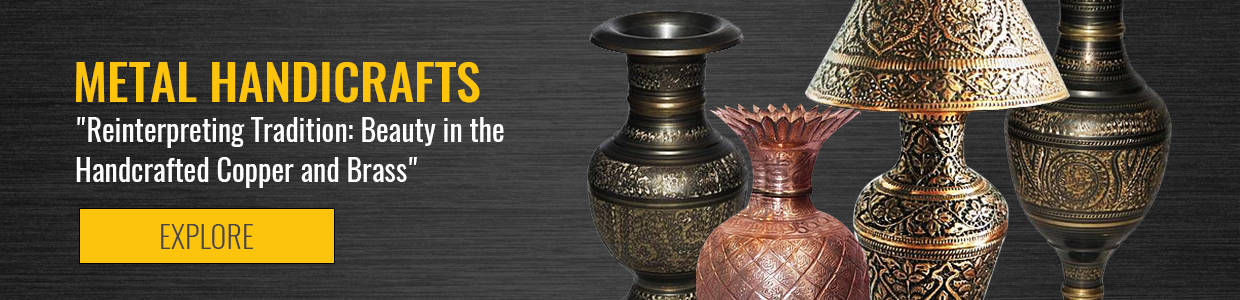## Metal Crafts

Brass and copper have been worked in the northern Pakistan lands ever since the Mughals invaded and were amazed by the skill of local artisans. Extremely intricate carving on brass and metal is done in Swat and old bazaars of Peshawar to create wondrous creations which can momentarily stun you with their craftsmanship.

.

X

View Filter

x
Clear all x

Rs. 1,000
Rs. 2,000
Rs. 1,200
Rs. 1,800
Rs. 1,700
Rs. 1,600
Rs. 4,500
Rs. 1,700
Rs. 1,200
Rs. 1,700
Rs. 1,700
Rs. 450
Rs. 1,200
Rs. 1,450
Rs. 1,550
Rs. 1,600
Rs. 700
Rs. 950
Rs. 1,550
Rs. 1,650
Rs. 1,350
Rs. 1,650
Rs. 1,650
Rs. 1,650
Rs. 800
Rs. 1,250
Rs. 1,450
Rs. 600
Rs. 1,850
Rs. 1,850
Rs. 1,650
Rs. 1,100
Rs. 950
Rs. 950
Rs. 950
Rs. 700
Rs. 900
Rs. 1,950
Rs. 1,850
Rs. 950
Rs. 8,000
Rs. 7,500
Rs. 9,950
Rs. 9,950
Rs. 7,000
Rs. 4,500
Rs. 4,500
Rs. 3,500
Rs. 3,500
Rs. 3,500
Rs. 9,000
Rs. 1,200
Rs. 1,200
Rs. 1,200
Rs. 1,200
Rs. 3,750
Rs. 1,350
Rs. 6,000
Rs. 2,160
Rs. 2,160
Rs. 950
Rs. 2,850
Rs. 2,850
Rs. 1,450
Rs. 1,450
Rs. 2,100
Rs. 2,310
Rs. 1,190
Rs. 770
Rs. 770
Rs. 2,100
Rs. 2,100
Rs. 2,100
Rs. 5,040
Rs. 770
Rs. 2,100
Rs. 4,200
Rs. 8,400
Rs. 1,190
Rs. 1,960
Rs. 2,310
Rs. 9,450
Rs. 9,450
Rs. 2,520
Rs. 2,310
Rs. 2,940
Rs. 3,780
Rs. 2,520
Rs. 5,880
Rs. 1,800
Rs. 1,800
Rs. 7,980
Rs. 7,980
Rs. 7,980
Rs. 7,980
Rs. 1,890
Rs. 8,820